# How to Check If Value Is In List in Excel

So, there are times when you would like to know that a value is in a list or not. We have done this using VLOOKUP. But we can do the same thing using COUNTIF function too. So in this article, we will learn how to check if a values is in a list or not using various ways.

#### Check If Value In Range Using COUNTIF Function

So as we know, using COUNTIF function in excel we can know how many times a specific value occurs in a range. So if we count for a specific value in a range and its greater than zero, it would mean that it is in the range. Isn’t it?

Generic Formula

=COUNTIF(range,value)>0

Range: The range in which you want to check if the value exist in range or not.

Value: The value that you want to check in the range.

Let’s see an example:

#### Excel Find Value is in Range Example

For this example, we have below sample data. We need a check-in the cell D2, if the given item in C2 exists in range A2:A9 or say item list. If it’s there then, print TRUE else FALSE.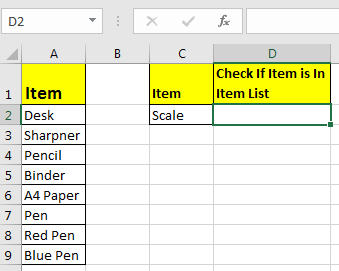Write this formula in cell D2:

=COUNTIF(A2:A9,C2)>0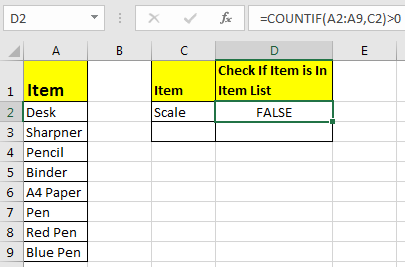Since C2 contains “scale” and it’s not in the item list, it shows FALSE. Exactly as we wanted. Now if you replace “scale” with “Pencil” in the formula above, it’ll show TRUE.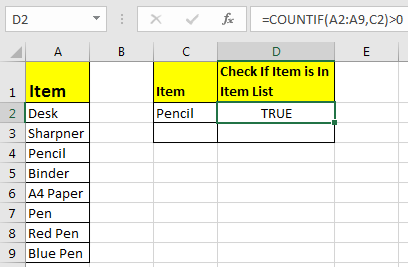Now, this TRUE and FALSE looks very back and white. How about customizing the output. I mean, how about we show, “found” or “not found” when value is in list and when it is not respectively.
Since this test gives us TRUE and FALSE, we can use it with IF function of excel.

Write this formula:

=IF(COUNTIF(A2:A9,C2)>0,"in List","Not in List")

You will have this as your output.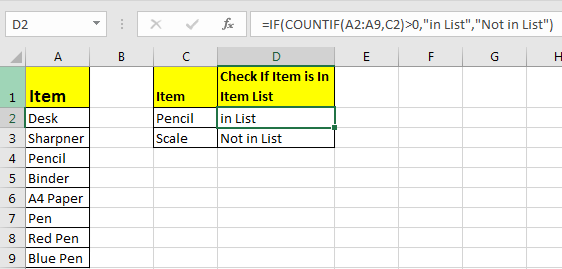What If you remove “>0” from this if formula?

=IF(COUNTIF(A2:A9,C2),"in List","Not in List")

It will work fine. You will have same result as above. Why? Because IF function in excel treats any value greater than 0 as TRUE.

#### How to check if a value is in Range with Wild Card Operators

Sometimes you would want to know if there is any match of your item in the list or not. I mean when you don’t want an exact match but any match.

For example, if in the above-given list, you want to check if there is anything with “red”. To do so, write this formula.

=IF(COUNTIF(A2:A9,"*red*"),"in List","Not in List")

This will return a TRUE since we have “red pen” in our list. If you replace red with pink it will return FALSE. Try it.
Now here I have hardcoded the value in list but if your value is in a cell, say in our favourite cell B2 then write this formula.

IF(COUNTIF(A2:A9,"*"&B2&"*"),"in List","Not in List")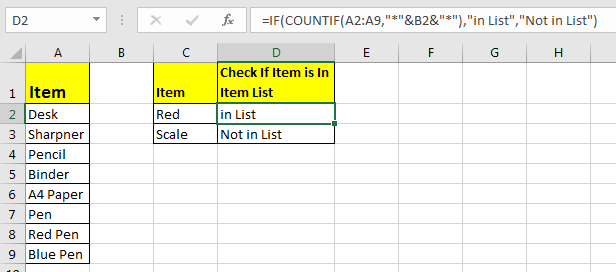There’s one more way to do the same. We can use the MATCH function in excel to check if the column contains a value. Let’s see how.

#### Find if a Value is in a List Using MATCH Function

So as we all know that MATCH function in excel returns the index of a value if found, else returns #N/A error. So we can use the ISNUMBER to check if the function returns a number.
If it returns a number ISNUMBER will show TRUE, which means it’s found else FALSE, and you know what that means.

Write this formula in cell C2:

=ISNUMBER(MATCH(C2,A2:A9,0))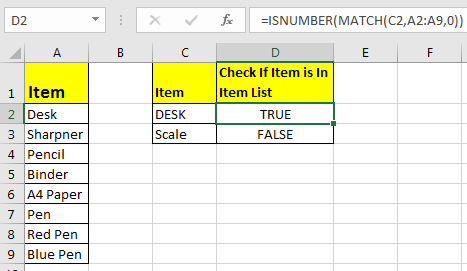The MATCH function looks for an exact match of value in cell C2 in range A2:A9. Since DESK is on the list, it shows a TRUE value and FALSE for SCALE.

So yeah, these are the ways, using which you can find if a value is in the list or not and then take action on them as you like using IF function. I explained how to find value in a range in the best way possible. Let me know if you have any thoughts. The comments section is all yours.

Related Articles:

How to Check If Cell Contains Specific Text in Excel

How to Check A list of Texts In String in Excel

How to take the Average Difference between lists in Excel

How to Get Every Nth Value From A list in Excel

Popular Articles:

50 Excel Shortcuts to Increase Your Productivity

How to use the VLOOKUP Function in Excel

How to use the COUNTIF function in Excel

How to use the SUMIF Function in Excel

1.